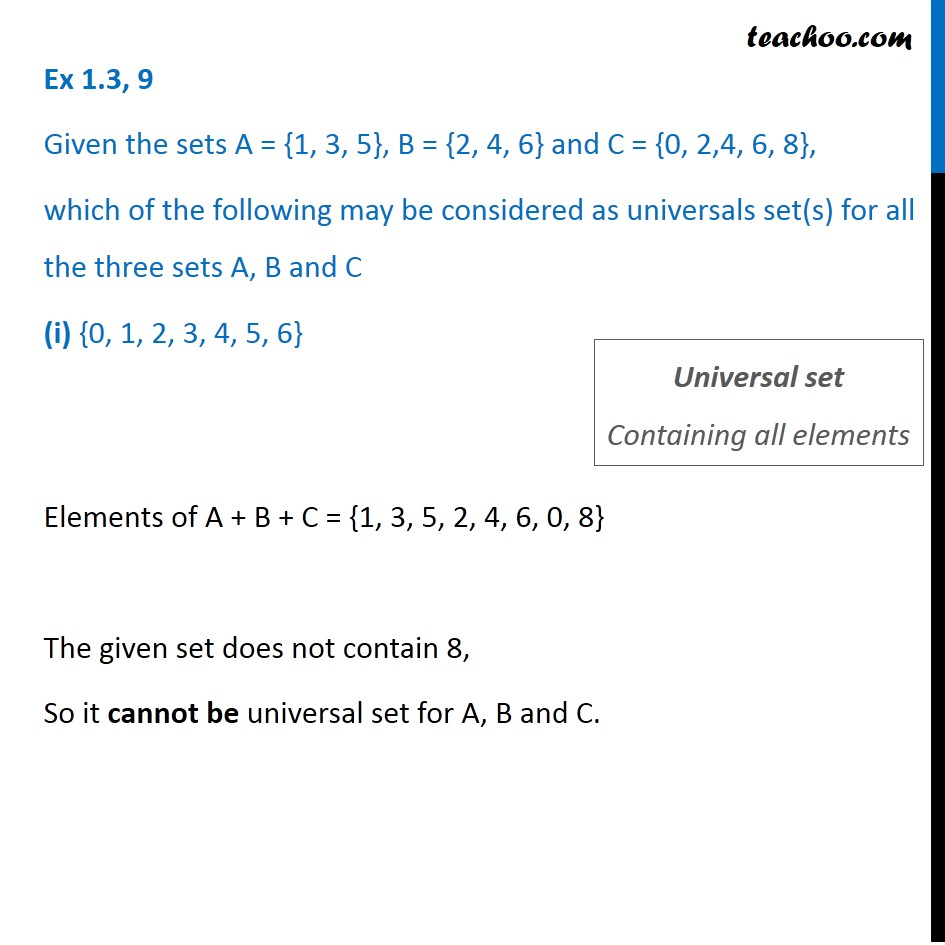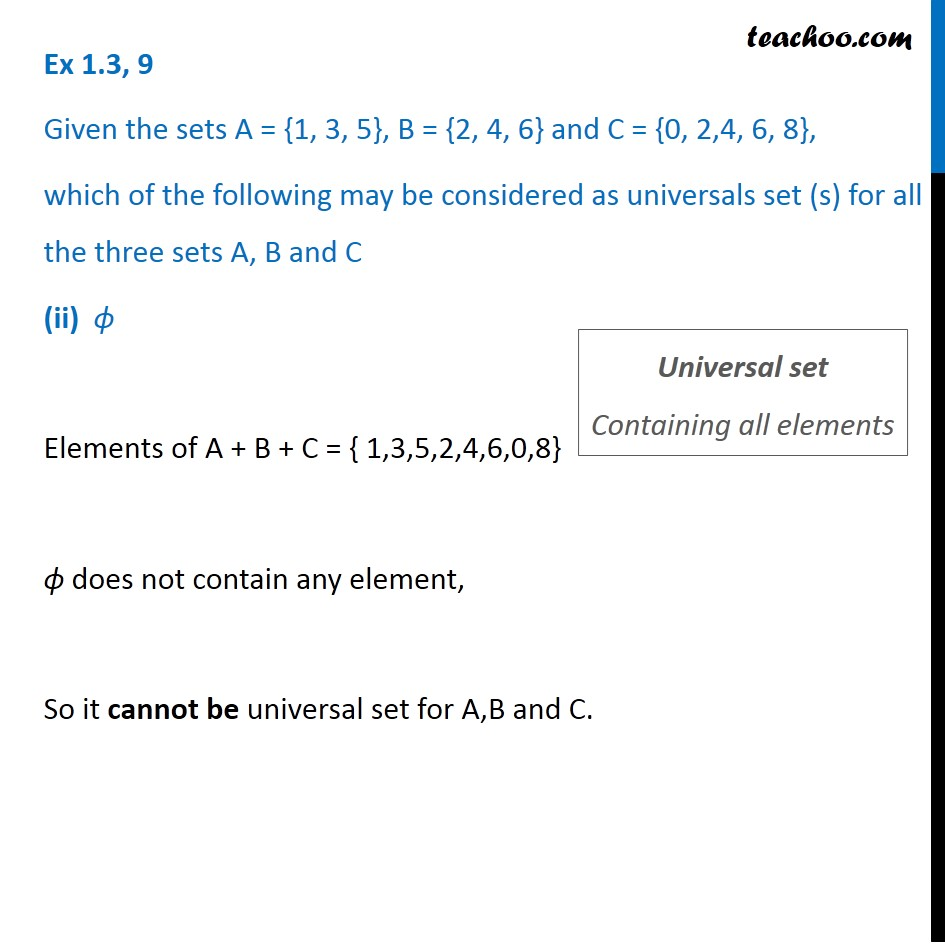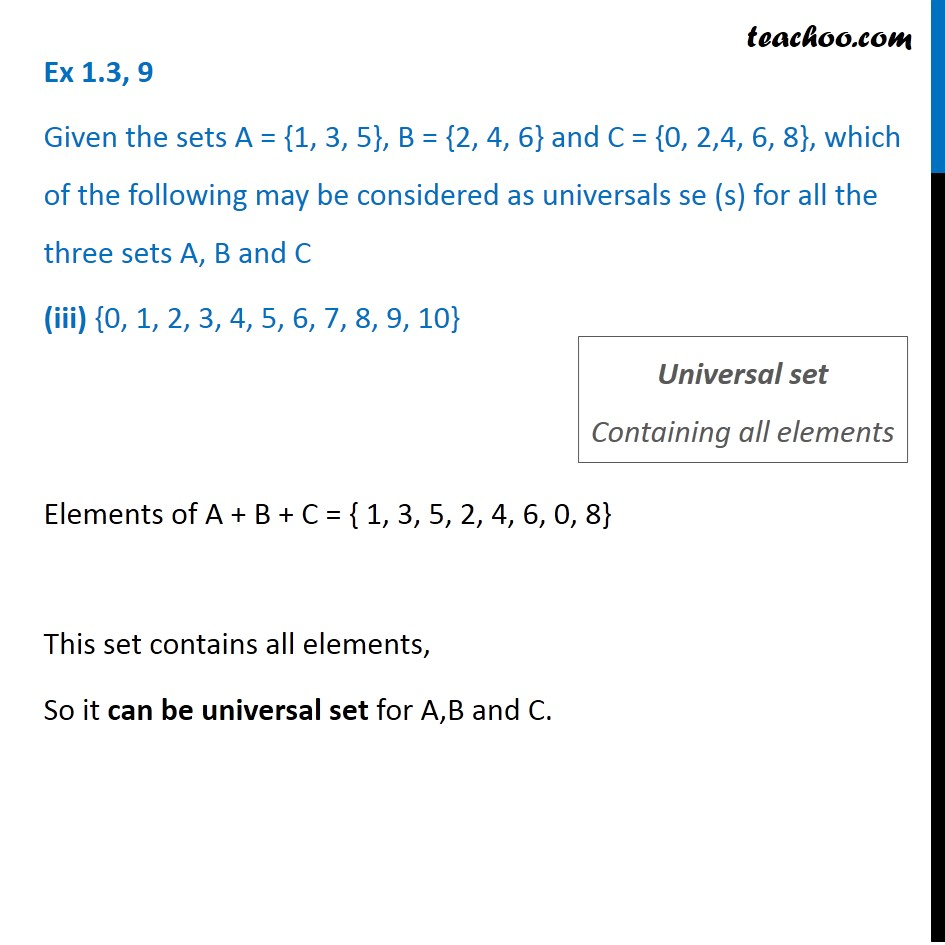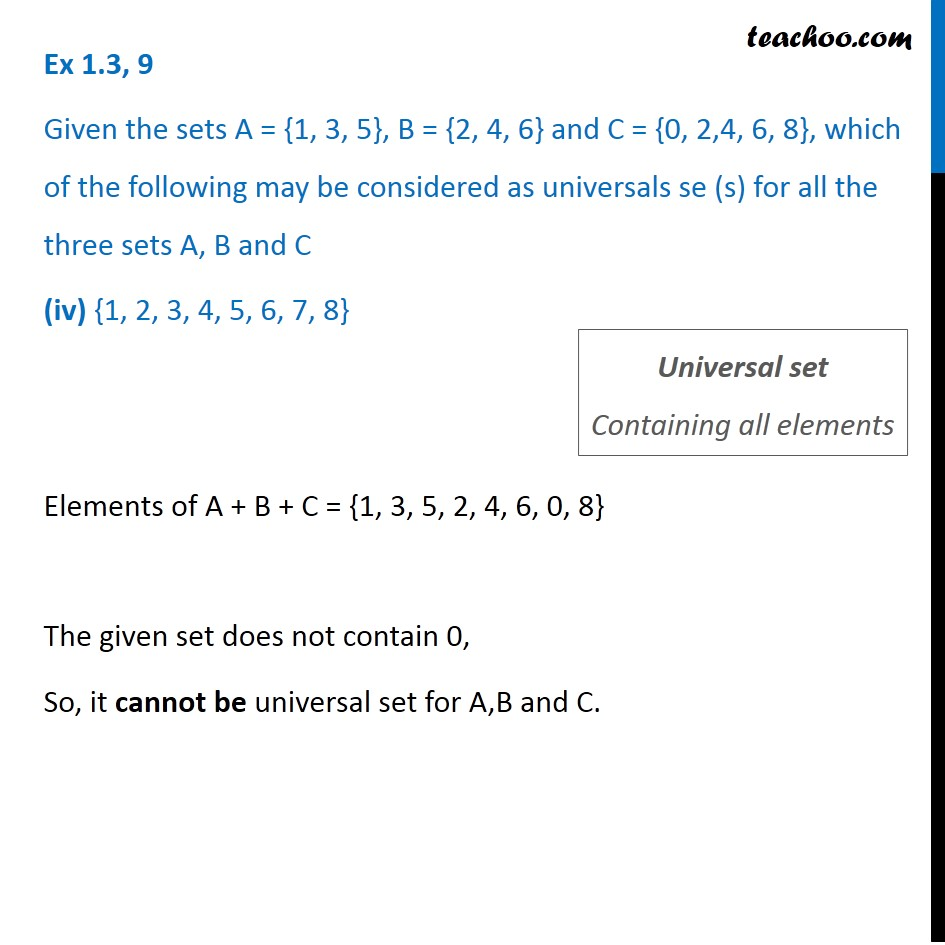Universal Set

Chapter 1 Class 11 Sets
Concept wiseLearn in your speed, with individual attention - Teachoo Maths 1-on-1 Class

### Transcript

Ex 1.3,9 Given the sets A = {1, 3, 5}, B = {2, 4, 6} and C = {0, 2,4, 6, 8}, which of the following may be considered as universals set(s) for all the three sets A, B and C (i) {0, 1, 2, 3, 4, 5, 6} Elements of A + B + C = { 1,3,5,2,4,6,0,8} The given set does not contain 8, so it cannot be universal set for A,B and C. Ex 1.3,9 Given the sets A = {1, 3, 5}, B = {2, 4, 6} and C = {0, 2,4, 6, 8}, which of the following may be considered as universals set (s) for all the three sets A, B and C (ii) Elements of A + B + C = { 1,3,5,2,4,6,0,8} does not contain any element, So it cannot be universal set for A,B and C. Ex 1.3,9 Given the sets A = {1, 3, 5}, B = {2, 4, 6} and C = {0, 2,4, 6, 8}, which of the following may be considered as universals se (s) for all the three sets A, B and C (iii) {0, 1, 2, 3, 4, 5, 6, 7, 8, 9, 10} Elements of A + B + C = { 1,3,5,2,4,6,0,8} This set contains all elements, So it can be universal set for A,B and C. Ex 1.3,9 Given the sets A = {1, 3, 5}, B = {2, 4, 6} and C = {0, 2,4, 6, 8}, which of the following may be considered as universals se (s) for all the three sets A, B and C (iv) {1, 2, 3, 4, 5, 6, 7, 8} Elements of A + B + C = { 1,3,5,2,4,6,0,8} The given set does not contain 0, So it cannot be universal set for A,B and C.